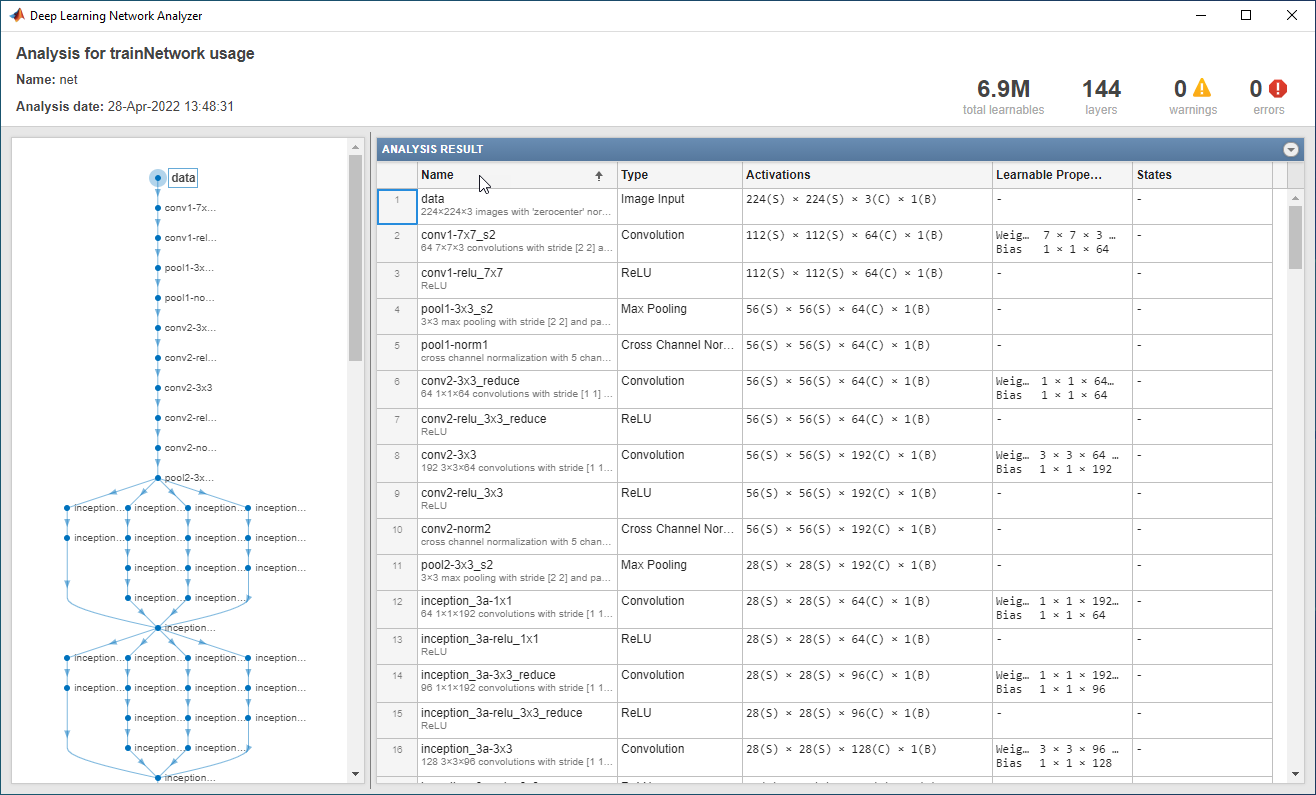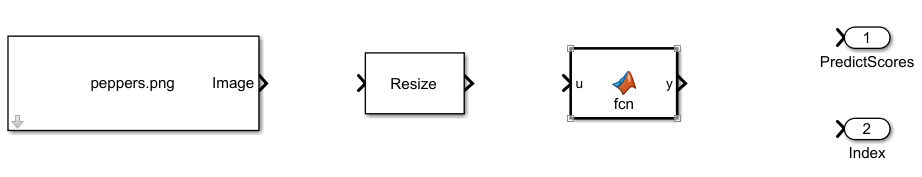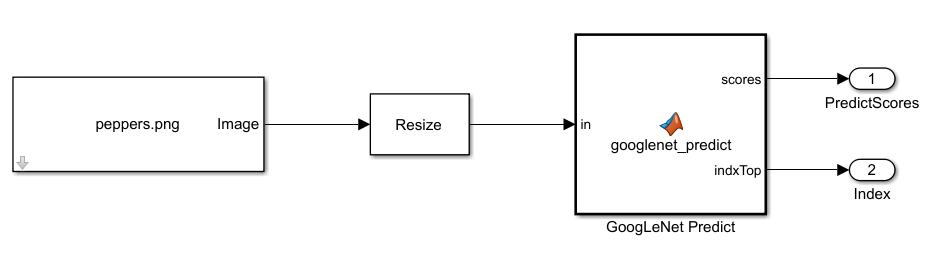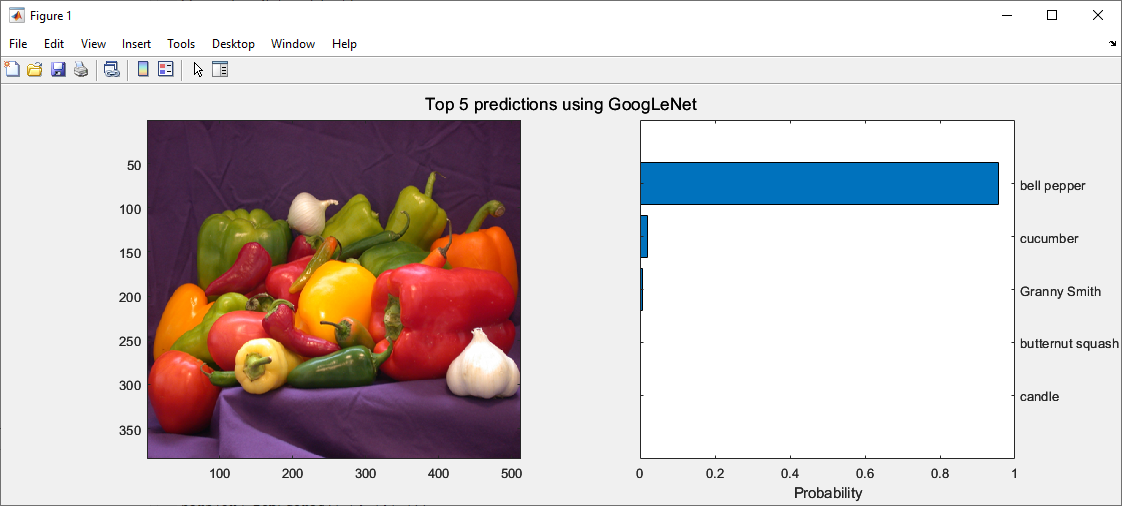## Generate Code for Deep Learning Networks Using MATLAB Function Block

You can generate optimized code for Simulink® models containing a variety of trained deep learning networks. You can implement the deep learning functionality in Simulink by using MATLAB Function blocks or by using blocks from the Deep Neural Networks library. When implementing deep learning functionality with MATLAB® Function blocks, use the `coder.loadDeepLearningNetwork` function to load a trained deep learning network and use the object functions of the network object to obtain the desired responses. You can configure the code generator to take advantage of the Intel® Math Kernel library for Deep Neural Networks (MKL-DNN). You can also generate generic C or C++ code that does not depend on third-party libraries. The generated code implements the deep convolutional neural network (CNN) by using the architecture, the layers, and parameters that you specify in a network object.

### Classify Images by Using GoogLeNet

GoogLeNet is a convolutional neural network that is 22 layers deep. The network has been trained on over a million images and can classify an image into 1000 object categories (such as a keyboard, coffee mug, pencil, and animals).The network takes an image as input, and then outputs a label for the object in the image together with the probabilities for each of the object categories. This example shows you how to perform simulation and generate C++ code for the pretrained GoogLeNet deep convolutional neural network and classify an image.

1. Load the pretrained GoogLeNet network. You can choose to load a different pretrained network for image classification. This function requires the Deep Learning Toolbox™ Model for GoogLeNet Network support package. If this support package is not installed, then the function provides a download link.

`net = googlenet;`

2. The object `net` contains the `DAGNetwork` object. Use the function to display an interactive visualization of the network architecture, to detect errors and issues in the network, and to display detailed information about the network layers. The layer information includes the sizes of layer activations and learnable parameters, the total number of learnable parameters, and the sizes of state parameters of recurrent layers.

`analyzeNetwork(net);`3. The image that you want to classify must have the same size as the input size of the network. For GoogLeNet, the size of the `imageInputLayer` is 224-by-224-by-3. The `Classes` property of the output `classificationLayer` contains the names the names of the classes learned by the network. View 10 random class names out of the total of 1000.

```classNames = net.Layers(end).Classes; numClasses = numel(classNames); disp(classNames(randperm(numClasses,10)))```
```speedboat window screen isopod wooden spoon lipstick drake hyena dumbbell strawberry custard apple ```

1. Create a Simulink model and insert a MATLAB Function block from the User-Defined Functions library.

2. Add an Image From File block from the Computer Vision Toolbox™ library and set the `File name` parameter to `peppers.png`.

3. Add a Resize block from the Computer Vision Toolbox library to the model. Set the Specify parameter of the Resize block to ```Number of output rows and columns``` and enter `[224 224]` as the value for Number of output rows and columns. This block resizes the input image to that of the input layer of the network.4. Double-click the MATLAB Function block. A default function signature appears in the MATLAB Function Block Editor.

5. Define a function called `googlenet_predict`, which implements the prediction entry-point function. The function header declares `in` as an argument to the `googlenet_predict` function, with `scores` and `indxTop` as the return value.

```function [scores,indxTop] = googlenet_predict(in) %#codegen persistent mynet; if isempty(mynet) mynet = coder.loadDeepLearningNetwork('googlenet'); end % pass in input predict_scores = predict(mynet,in); [scores,indx] = sort(predict_scores, 'descend'); indxTop = indx(1:5); ```

A persistent object `mynet` loads the `DAGNetwork` object. At the first call to the entry-point function, the persistent object is constructed and set up. On subsequent calls to the function, the same object is reused to call `predict` on inputs, avoiding reconstructing and reloading the network object.

You can use the `activations` (Deep Learning Toolbox) method to network activations for a specific layer. For example, the following line of code returns the network activations for the layer specified in `layerIdx`.

```out = activations(mynet,in,layerIdx,'OutputAs','Channels'); ```

You can use the `classify` (Deep Learning Toolbox) method to predict class labels for the image data in `in` using the trained network `mynet`.

```[out,scores] = classify(mynet,in); ```

For LSTM networks, you can use the `predictAndUpdateState` (Deep Learning Toolbox) and `resetState` (Deep Learning Toolbox) methods.

6. Open the block parameters of the MATLAB Function block. On the Code Generation tab, select ```Reusable function``` for Function packaging.

7. Connect these blocks as shown in the diagram. Save the model as `googlenetModel`.### Simulate Model

1. Open the Configuration Parameters dialog box. Select the Solver pane. Set the Type parameter to `Fixed-step`. This setting maintains a constant (fixed) step size, which is required for code generation.

2. Select the Simulation Target pane. Set the Language to `C++`.

3. On the Simulation Target pane, set the Target Library parameter in the Deep learning group to `MKL-DNN`.

4. Click OK to save and close the Configuration Parameters dialog box.

5. To build and simulate the model, use this command:

`out = sim('googlenetModel');`

6. Display the top five predicted labels and their associated probabilities as a histogram. Because the network classifies images into so many object categories, and many categories are similar, it is common to consider the top-five accuracy when evaluating networks. The network classifies the image as a bell pepper with a high probability.

```im = imread('peppers.png'); classNamesTop = classNames(out.yout{2}.Values.Data(:,:,1)) h = figure; h.Position(3) = 2*h.Position(3); ax1 = subplot(1,2,1); ax2 = subplot(1,2,2); image(ax1,im); barh(ax2,out.yout{1}.Values.Data(1,5:-1:1,1)) xlabel(ax2,'Probability') yticklabels(ax2,classNamesTop(5:-1:1)) ax2.YAxisLocation = 'right'; sgtitle('Top 5 predictions using GoogLeNet') ```### Generate Code

1. Open the Simulink Coder or Embedded Coder app. The C Code tab opens.

2. In the Configuration Parameters dialog box, if you have a Simulink Coder™ license, set the System target file to `grt.tlc`. If you have Embedded Coder®, set the System target file parameter to `ert.tlc`.

3. Set the Language to `C++`.

4. Select Generate code only.

5. On the Code Generation > Interface pane, set the Target Library in the Deep learning group to `MKL-DNN`.

6. Clear the MAT-file logging parameter.

7. Click OK to save and close the Configuration Parameters dialog box.

8. On the C++ Code app, click Generate Code.